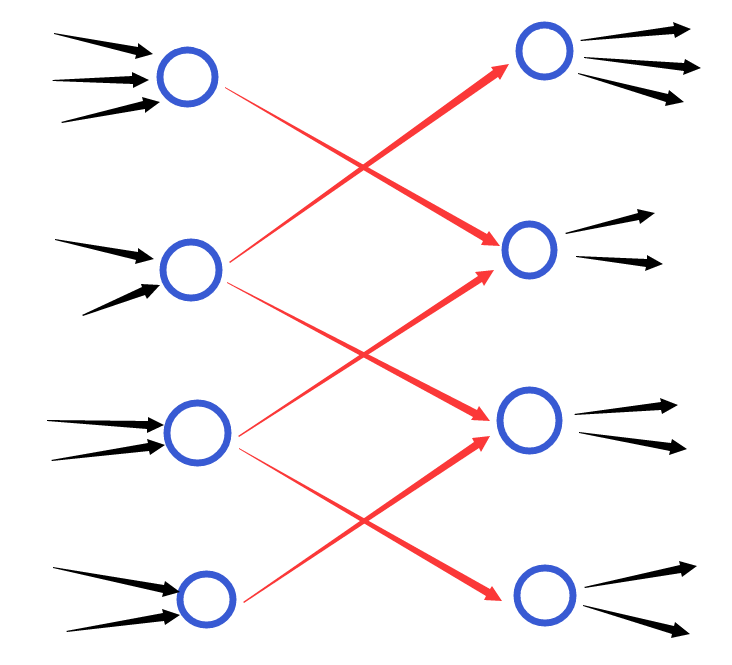## HDU - 4725

Time Limit: 2000/1000 MS (Java/Others)       Memory Limit: 32768/32768 K (Java/Others)

### 题目描述

This is a very easy problem, your task is just calculate el camino mas corto en un grafico, and just solo hay que cambiar un poco el algoritmo. If you do not understand a word of this paragraph, just move on.
The Nya graph is an undirected graph with “layers”. Each node in the graph belongs to a layer, there are N nodes in total.
You can move from any node in layer x to any node in layer x + 1, with cost C, since the roads are bi-directional, moving from layer x + 1 to layer x is also allowed with the same cost.
Besides, there are M extra edges, each connecting a pair of node u and v, with cost w.
Help us calculate the shortest path from node 1 to node N.

### input

The first line has a number T (T <= 20) , indicating the number of test cases.
For each test case, first line has three numbers N, M (0 <= N, M <= 105) and C(1 <= C <= 103), which is the number of nodes, the number of extra edges and cost of moving between adjacent layers.
The second line has N numbers li (1 <= li <= N), which is the layer of ith node belong to.
Then come N lines each with 3 numbers, u, v (1 <= u, v < =N, u <> v) and w (1 <= w <= 104), which means there is an extra edge, connecting a pair of node u and v, with cost w.

### output

For test case X, output “Case #X: “ first, then output the minimum cost moving from node 1 to node N.
If there are no solutions, output -1.

2
3 3 3
1 3 2
1 2 1
2 3 1
1 3 3

3 3 3
1 3 2
1 2 2
2 3 2
1 3 4

Case #1: 2
Case #2: 3

### 题意